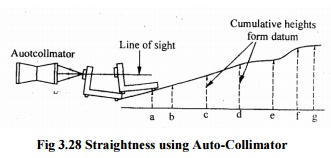Home | | Metrology and Measurements | Straightness Measurement

# Straightness Measurement

A line is said to be straight over a given length, if the variation of the distance of its from two planes perpendicular to each other and parallel to the general direction of the line remains within the specified tolerance limits.

STRAIGHTNESS MEASUREMENT

A line is said to be straight over a given length, if the variation of the distance of its from two planes perpendicular to each other and parallel to the general direction of the line remains within the specified tolerance limits. The tolerance on the straightness of a line is defined as the maximum deviation in relation to the reference straight line joining the two extremities of the line to be checkedStraight edge

A straight edge is a measuring tool which consists of a length of a length of a steel of narrow and deep section in order to provide resistance to bending in the plane of measurement without excessive weight. For checking the straightness of any surface, the straight edge is placed over the surface and two are viewed against the light, which clearly indicate the straightness. The gap between the straight edge and surface will be negligibly small for perfect surfaces. Straightness is measured by observing the colour of light by diffraction while passing through the small gap. If the colour of light be red, it indicates a gap of 0.0012 to 0.0075mm. A more accurate method of finding the straightness by straight edges is to place it in equal slip gauges at the correct point for minimum deflection and to measure the uniformity of space under the straight edge with slip gauges.

Test for straightness by using spirit level and Autocollimator

The straightness of any surface could be determined by either of these instruments by measuring the relative angular positions of number of adjacent sections of the surface to be tested. First straight line is drawn on the surface then it is divided into a number of sections the length of each section being equal to the length of sprit level base or the plane reflector’ s baseThebasesinofthe casespiritlevel blockof orauto c reflector are fitted with two feet so that only feet have line contact with the surface and the surface of base does not touch the surface to he tested. The angular division obtained is between the specified two points. Length of each section must be equal to distance between the centerlines of two feet. The special level can be used only for the measurement of straightness of horizontal surfaces while auto-collimator can be used on surfaces are any plane. In case of spirit level, the block is moved along the line equal to the pitch distance between the centerline of the feet and the angular variation of the direction of block. Angular variation can be determined in terms of the difference of height between two points by knowing the least count of level and length of the base.Fig 3.28 Straightness using Auto-Collimator

In case of autocollimator the instrument is placed at a distance of 0.5 to 0.75m from the surface to be tested. The parallel beam from the instrument is projected along the length of the surface to be tested. A block fixed on two feet and fitted with a plane vertical reflector is placed on the surface and the reflector face is facing the instrument. The image of the cross wires of the collimator appears nearer the center of the field and for the complete movement of reflector along the surface straight line the image of cross wires will appear in the field of eyepiece. The reflector is then moved to the other end of the surface in steps equal to. The center distance between the feet and the tilt of the reflector is noted down in second from the eyepiece.

Study Material, Lecturing Notes, Assignment, Reference, Wiki description explanation, brief detail
Mechanical : Metrology and Measurements : Thread Measurement : Straightness Measurement |